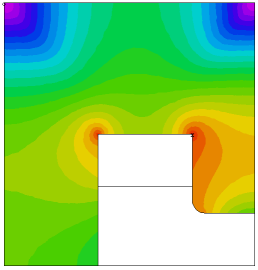﻿ Sample Problems > Applications > Magnetism > saturation

saturation

Navigation:  Sample Problems > Applications > Magnetism >

saturation{ SATURATION.PDE

A NONLINEAR MAGNETOSTATIC PROBLEM

This example considers the problem of determining the magnetic vector

potential A in a cyclotron magnet.

The problem domain consists of

1) a ferromagnetic medium - the magnet core,

2) the surrounding air medium,

3) a current-carrying copper coil.

According to Maxwell's equations,

curl H = J                      (1)

div B = 0                       (2)

with

B = mu*H

where B is the magnetic flux density

H is the magnetic field strength

J is the electric current density

and  mu is the magnetic permeability of the material.

Maxwell's equations can be satisfied if we introduce a magnetic vector

potential A such that

B = curl A

therefore

curl( (curl A)/mu ) = J

This equation is usually supplemented with the Coulomb Gauge condition

div A = 0.

In most common 2D applications, magnet designers assume either

1) that the magnet is sufficiently long in the z direction or

2) that the magnet is axi-symmetric.

In the first instance the current is assumed to flow parallel to the z axis,

and in the latter it flows in the azimuthal direction.  Under these conditions,

only the z or the azimuthal component of A is present. (Henceforth, we will

simply refer to this component as A).

In the Cartesian case, the magnetic induction B takes the simple form,

B = (dy(A), -dx(A), 0)

and the magnetic field is given by

H = (dy(A)/mu, -dx(A)/mu, 0).

We can integrate equation (1) over the problem domain using the curl analog

of the Divergence Theorem, giving

Integral(curl(H))dV = Integral(n x H)dS

where dS is a differential surface area on the bounding surface of any region,

and n is the outward surface normal unit vector.

Across interior boundaries between regions of different material properties,

FlexPDE assumes cancellation of the surface integrals from the two sides of the

boundary.  This implies continuity of (n X H).

At exterior boundaries, the same theorem defines the natural boundary condition

to be the value of (n x H).

For the present example, let us define the permeability mu by the

expression

mu =  1                                                    in the air and the coil

mu =  mumax/(1+C*grad(A)^2) + mumin     in the core

where C = 0.05 gives a behaviour similar to transformer steel.

We assume a symmetry plane along the X-axis, and impose the boundary value

A = 0 along the remaining sides.

The core consists of a "C"-shaped region enclosing a rectangular coil region.

The source J is zero everywhere except in the coil, where it is defined by

J = - (4*pi/10)*amps/area

Note:

This example uses scaled units.  It is important for the user to validate

the dimensional consistency of his formulation.

}

 Title "A MAGNETOSTATIC PROBLEM" Select   { Since the nonlinearity in this problem is driven      by the GRADIENT of the system variable, we require      a more accurate resolution of the solution: }    errlim = 1e-4     Variables    A Definitions    rmu = 1    rmu0 = 1    mu0core = 5000    mu1core = 200    mucore = mu0core/(1+0.05*grad(A)^2) + mu1core    rmucore = 1/mucore    S = 0    current = 2    y0 = 8 Initial Value   { In nonlinear problems, a good starting value      is sometimes essential for convergence }    A = current*(400-(x-20)^2-(y-20)^2) Equations    A : curl(rmu*curl(A)) = SBoundaries

Region 1           { The IRON core }

rmu = rmucore                rmu0 = 1/mu0core

start(0,0)

natural(A) = 0       line to (40,0)

value(A) = 0   line to (40,40) to (0,40) to close

Region 2           { The AIR gap }

rmu = 1

start (15,0) line to (40,0) to (40,y0) to (32,y0)

arc (center=32,y0+2) to (30,y0+2)

{ short boundary segments force finer gridding: }

line to (30,19.5) to (30,20) to (29.5,20)

to (15.5,20) to (15,20) to close

Region 3           { The COIL }

S = current

rmu = 1

start (15,12) line to (30,12) to (30,20) to (15,20) to close

Monitors

contour(A)

Plots

grid(x,y)

vector(dy(A),-dx(A)) as "FLUX DENSITY B"

vector(dy(A)*rmu, -dx(A)*rmu) as "MAGNETIC FIELD H"

contour(A) as "Az MAGNETIC POTENTIAL"

surface(A) as "Az MAGNETIC POTENTIAL"

contour(rmu/rmu0) painted as "Saturation: mu0/mu"

End